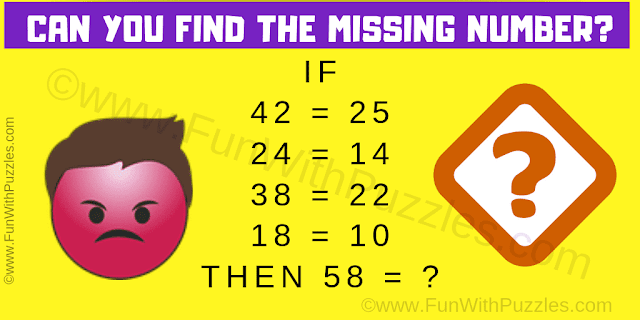## Friday, December 6, 2019

This Maths Logic Puzzle will twist your brain. In this logic number puzzle, you are shown some number equation. These number equations are correct logical and follow some logical or mathematical pattern. Your challenge is to decode this logical pattern used in these number equations and then find the value of the missing number. Let us see if you can solve this logic puzzle in maths?Can you solve this logic puzzle?
The answer to this "Logic Puzzle in Maths", can be viewed by clicking on the answer button.